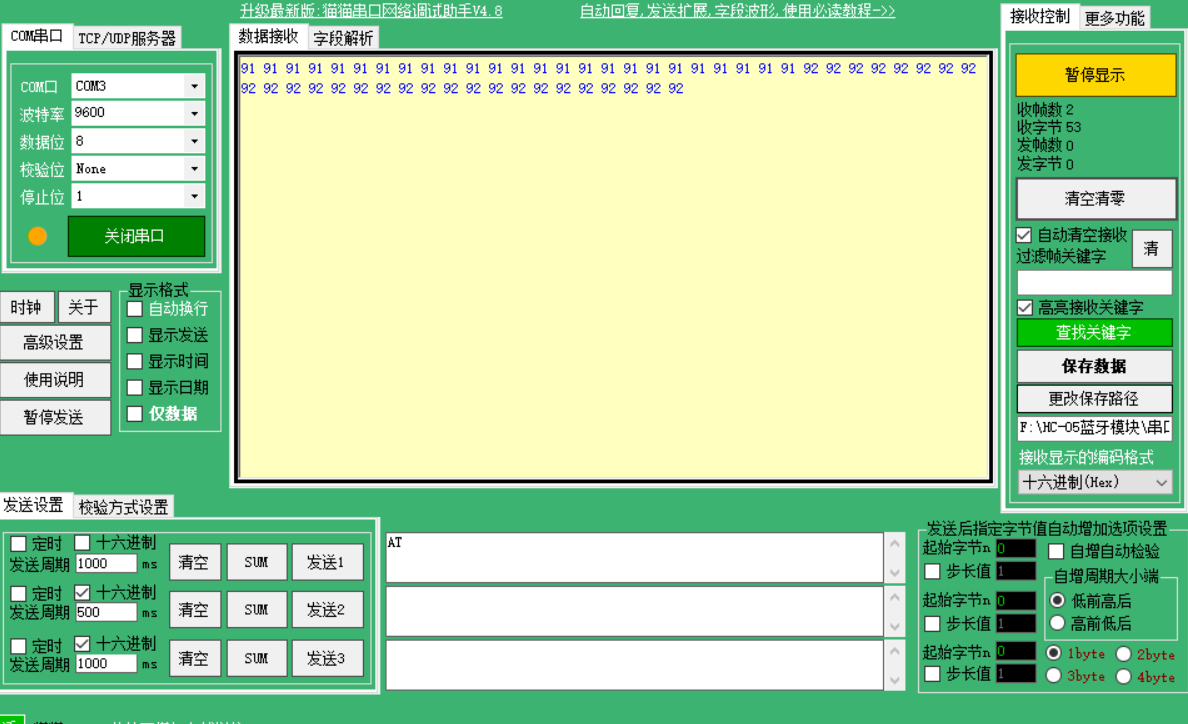STC89C52单片机接蓝牙模块向PC发消息显示乱码怎么解决。

AT指令设置已经成功了，两个蓝牙可以配对上，但是传消息出现乱码。``````#include <reg51.h>
typedef unsigned int u16;

sbit K3=P3^2;
sbit K4=P3^3;
unsigned char RX;

void InitUART(void)
{
TMOD = 0x20;
SCON = 0x40;
TH1 = 0xFD;
TL1 = TH1;
PCON = 0x00;
TR1 = 1;
}
void delay(u16 i)
{
while(i--);
}

void SendOneByte(unsigned char c)
{
SBUF =c;
while(!TI);
TI = 0;
}

void main(void)
{
InitUART();
while(1)
{
if(K3==0)
{
delay(500);     //消抖
if(K3==0)
SendOneByte(0X31);    //发送控制字符‘1’
}
if(K4==0)
{
delay(500);
if(K4==0)
SendOneByte(0X32);   //发送‘2’
}
}
}

``````

1个回答